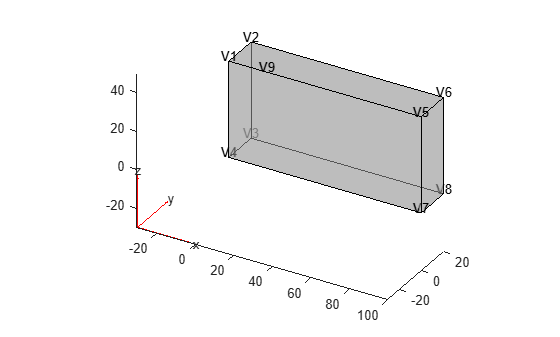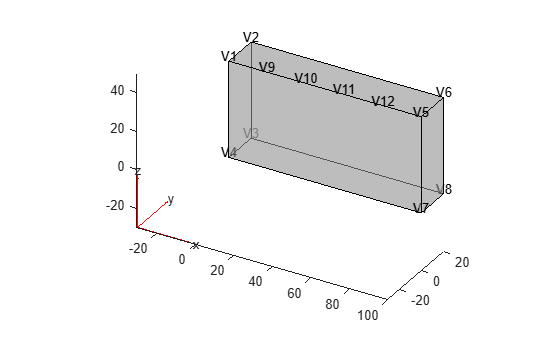Add a vertex on a geometry boundary

## Syntax

``VertexID = addVertex(g,'Coordinates',Coords)``

## Description

example

````VertexID = addVertex(g,'Coordinates',Coords)` adds a new isolated vertex at the point with coordinates `Coords` to a boundary of the geometry `g`. To add several vertices simultaneously, specify `Coords` as an `N`-by-2 matrix for a 2-D geometry or an `N`-by-3 matrix for a 3-D geometry. Here, `N` is the number of new points.If a point with the specified coordinates is slightly offset (within an internally specified tolerance) from a geometry boundary, `addVertex` approximates it to a point on the boundary. If a vertex already exists at the specified location, `addVertex` returns the ID of the existing vertex instead of creating one.```

## Examples

collapse all

Use `addVertex` to add a single vertex and multiple vertices on a side of a block geometry.

Create a PDE model.

`model = createpde();`

Import the geometry.

`g = importGeometry(model,'Block.stl');`

Plot the geometry and display the vertex labels.

`pdegplot(g, 'VertexLabels', 'on','FaceAlpha',0.5)`Add a vertex on the edge of a block.

`VertexID = addVertex(g,'Coordinates',[20 0 50])`
```VertexID = 9 ```

Plot the geometry and display the vertex labels.

`pdegplot(g, 'VertexLabels', 'on','FaceAlpha',0.5)`Add three more vertices on the same edge of the block.

```V = ([40 0 50; 60 0 50; 80 0 50]); VertexIDs = addVertex(g,'Coordinates',V)```
```VertexIDs = 3×1 10 11 12 ```

Plot the geometry and display the vertex labels.

`pdegplot(g, 'VertexLabels', 'on','FaceAlpha',0.5)`Add a vertex at the corner of the block. Since there is already a vertex at the corner, `addVertex` does not create a new vertex, but returns the ID of the existing vertex.

`VertexID = addVertex(g,'Coordinates',[100 0 50])`
```VertexID = 5 ```

## Input Arguments

collapse all

Geometry, specified as a `DiscreteGeometry` object.

Coordinates of a new vertex, specified as an `N`-by-2 or `N`-by-3 numeric matrix for a 2-D or 3-D geometry, respectively. Here, `N` is the number of new vertices.

Example: `'Coordinates',[0;0;1]`

Data Types: `double`

## Output Arguments

collapse all

Vertex ID, returned as a row vector of positive numbers. Each number represents a vertex ID. When you add a new vertex to a geometry with `N` vertices, the ID of the added vertex is `N + 1`. If a vertex already exists at the specified location, `addVertex` returns the ID of the existing vertex.

## Version History

Introduced in R2019b